# 8  Making maps with Python

## 8.1 Prerequisites

Let’s import the required packages:

import matplotlib.pyplot as plt
import geopandas as gpd
import rasterio
import rasterio.plot
import contextily as cx
import folium

and load the sample data for this chapter:

nz = gpd.read_file('data/nz.gpkg')
nz_elev = rasterio.open('data/nz_elev.tif')
tanzania_buf = tanzania.to_crs(32736).buffer(50000).to_crs(4326)
tanzania_neigh = gpd.read_file('data/world.gpkg', mask=tanzania_buf)

## 8.2 Introduction

A satisfying and important aspect of geographic research is communicating the results. Map making—the art of cartography—is an ancient skill that involves communication, intuition, and an element of creativity. In addition to being fun and creative, cartography also has important practical applications. A carefully crafted map can be the best way of communicating the results of your work, but poorly designed maps can leave a bad impression. Common design issues include poor placement, size and readability of text and careless selection of colors, as outlined in the style guide of the Journal of Maps. Furthermore, poor map making can hinder the communication of results (Brewer 2015, add citation…):

Amateur-looking maps can undermine your audience’s ability to understand important information and weaken the presentation of a professional data investigation. Maps have been used for several thousand years for a wide variety of purposes. Historic examples include maps of buildings and land ownership in the Old Babylonian dynasty more than 3000 years ago and Ptolemy’s world map in his masterpiece Geography nearly 2000 years ago (Talbert 2014, add citation…).

Map making has historically been an activity undertaken only by, or on behalf of, the elite. This has changed with the emergence of open source mapping software such as mapping packages in Python, R, and other languages, and the “print composer” in QGIS which enable anyone to make high-quality maps, enabling “citizen science”. Maps are also often the best way to present the findings of geocomputational research in a way that is accessible. Map making is therefore a critical part of geocomputation and its emphasis not only on describing, but also changing the world.

Basic static display of vector layers in Python is done with the .plot method or the rasterio.plot.show function, for vector layers and rasters, as we saw in Sections Section 1.2.2 and Section 1.3.2, respectively. Other, more advaned uses of these methods, were also encountered in later chapters, when demonstrating the various outputs we got. In this chapter, we provide a comprehensive summary of the most useful workflows of these two methods for creating static maps (Section 8.3). Then, we move on to elaborate on the .explore method for creating interactive maps, which was also briefly introduced earlier (Section 1.2.2).

## 8.3 Static maps

Static maps are the most common type of visual output from geocomputation. Standard formats include .png and .pdf for raster and vector outputs, respectively. Static maps can be easily shared and viewed (whether digitally or in print), however they can only convey as much information as a static image can. Interactive maps provide much more flexibilty in terms of user experience and amout of information, however they often require more work to design and effectively share.

Let’s move on to the basics of static mapping with Python.

### 8.3.1 Minimal example

A vector layer (GeoDataFrame) or a geometry column (GeoSeries) can be displayed using their .plot method (Section 1.2.2). A minimal example of a vector layer map is obtained using .plot with nothing but the defaults (Figure 8.1):

nz.plot();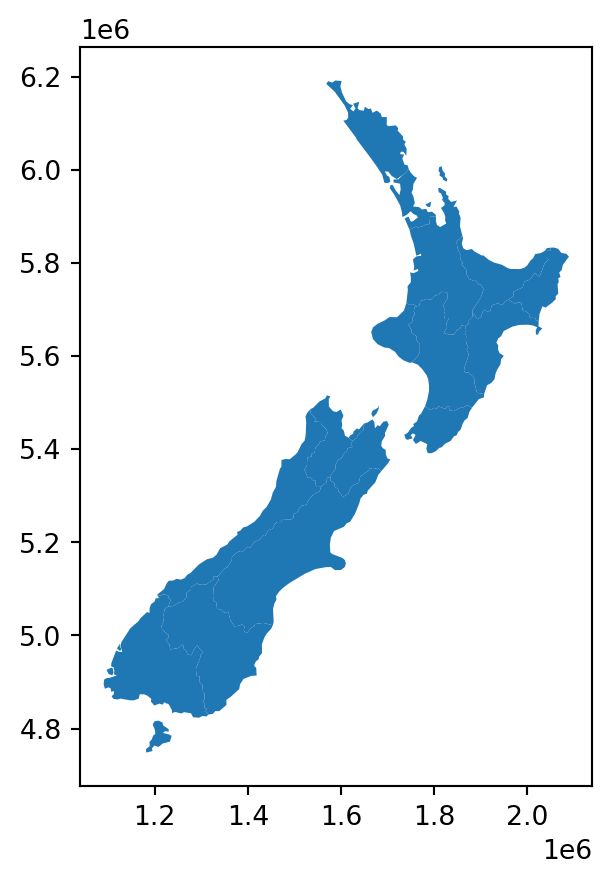Figure 8.1: Minimal example of a static vector layer plot with .plot

A rasterio raster file connection, or a numpy ndarray, can be displayed using rasterio.plot.show (Section 1.3.2). Here is a minimal example of a raster plot (Figure 8.2):

rasterio.plot.show(nz_elev);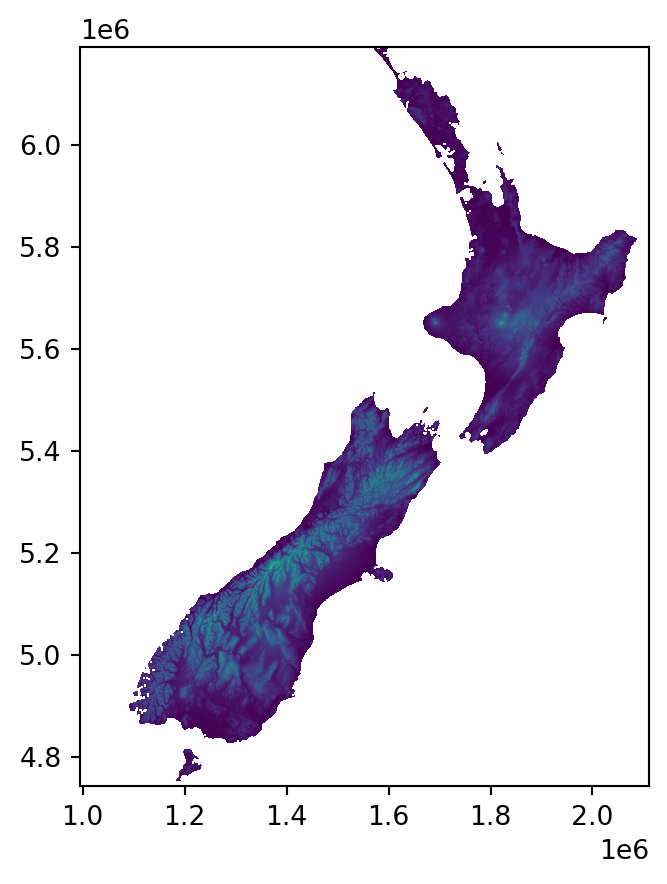Figure 8.2: Minimal example of a static raster plot with rasterio.plot.show

### 8.3.2 Styling

The most useful visual properties of the geometries, that can be specified in .plot, include color, edgecolor, and markersize (for points) (Figure 8.3):

nz.plot(color='grey');
nz.plot(color='none', edgecolor='blue');
nz.plot(color='grey', edgecolor='blue');

And here is an example of using markersize to get larger points (Figure 8.4). This example also demonstrated how to control the overall figure size, such as $$4 \times 4$$ $$in$$ in this case:

fig, ax = plt.subplots(figsize=(4,4))
nz_height.plot(markersize=100, ax=ax);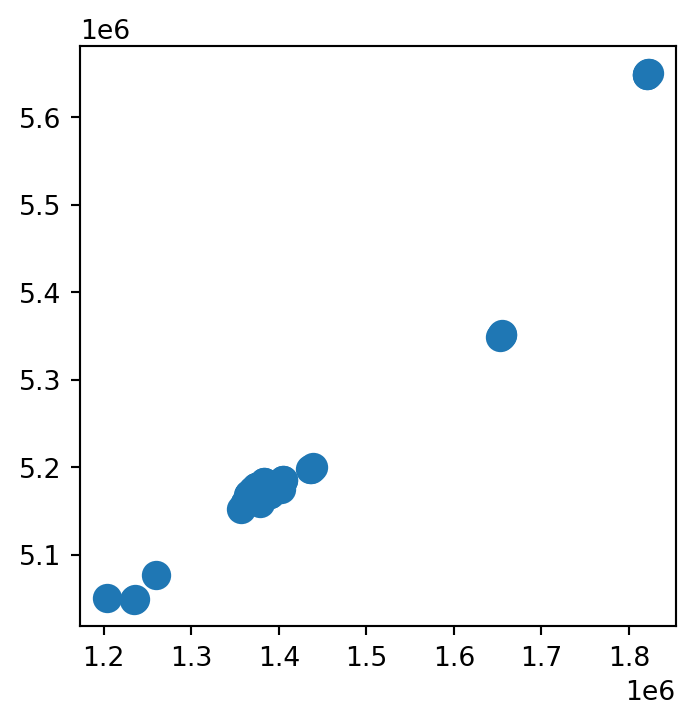Figure 8.4: Setting markersize in a static map of a vector layer

### 8.3.3 Symbology

We can set symbology in a .plot using the following parameters:

• column—A column name
• legend—Whether to show a legend
• cmap—Color map

For example, here we plot the polygons colored according to the 'Median_income' attribute (Figure 8.5):

nz.plot(column='Median_income', legend=True);

The default color scale which you see in Figure 8.5 is cmap='viridis'. However, the cmap (“color map”) argument can be used to specify any of countless other color scales. A first safe choice is often the ColorBrewer collection of color scales, specifically chosen for mapping uses. Any color scale can be reversed, using the _r suffic. Finally, other color scales are available, see the matplotlib colormaps article for details. The following code sections demonstrates these color scale specifications (Figure 8.6):

nz.plot(column='Median_income', legend=True, cmap='Reds');
nz.plot(column='Median_income', legend=True, cmap='Reds_r');
nz.plot(column='Median_income', legend=True, cmap='spring');

Categorical symbology is also supported, such as when column points to a string attribute. For example, the following expression sets symbology according to the 'Island' column. In this case, it makes sense to use a qualitative color scale, such as 'Set1' from ColorBrewer (Figure 8.7):

nz.plot(column='Island', legend=True, cmap='Set1');

In case the legend interferes with the contents (such as in Figure 8.7), we can modify the legend position as follows (Figure 8.8):

nz.plot(column='Island', legend=True, cmap='Set1', legend_kwds={'loc': 4});

The rasterio.plot.show function, based on matplotlib as well, supports the same kinds of cmap arguments. For example (Figure 8.9):

rasterio.plot.show(nz_elev, cmap='BrBG');
rasterio.plot.show(nz_elev, cmap='BrBG_r');
rasterio.plot.show(nz_elev, cmap='nipy_spectral');

Unfortunately, there is no built-in option to display a legend in rasterio.plot.show. The following workaround, going back to matplotlib methods, can be used to acheive it instead (Figure 8.10):

fig, ax = plt.subplots()
rasterio.plot.show(nz_elev, cmap='BrBG', ax=ax);
fig.colorbar(i, ax=ax);

### 8.3.4 Layers

To display more than one layer in the same plot, we need to:

• store the first plot in a variable (e.g., base), and
• pass it as the ax argument of any subsequent plot(s) (e.g., ax=base).

For example, here is how we can plot nz and nz_height together (Figure 8.11):

base = nz.plot(color='none')
nz_height.plot(ax=base, color='red');

We can combine the rasters and vector layers inthe same plot as well, which we already used earlier when explaining masking and cropping (Figure 5.2). We need to initialize a plot with fig,ax=plt.subplots(), then pass ax to any of the separate plots, making them appear together.

For example, Figure 8.12 demonstrated plotting a raster with increasingly complicated additions:

• The left panel shows a raster (New Zealand elevation) and a vector layer (New Zealand administrative division)
• The center panel shows the raster with a buffer of 22.2 $$km$$ around the dissolved administrative borders, representing New Zealand’s territorial waters (see Section 3.4.6)
• The right panel shows the raster with two vector layers: the territorial waters (in red) and elevation measurement points (in yellow)
# Raster + vector layer
fig, ax = plt.subplots(figsize=(5, 5))
rasterio.plot.show(nz_elev, ax=ax)
nz.to_crs(nz_elev.crs).plot(ax=ax, facecolor='none', edgecolor='red');

# Raster + computed vector layer
fig, ax = plt.subplots(figsize=(5, 5))
rasterio.plot.show(nz_elev, ax=ax)
gpd.GeoSeries(nz.unary_union, crs=nz.crs) \
.to_crs(nz_elev.crs) \
.buffer(22200) \
.boundary \
.plot(ax=ax, color='red');

# Raster + two vector layers
fig, ax = plt.subplots(figsize=(5, 5))
rasterio.plot.show(nz_elev, ax=ax)
gpd.GeoSeries(nz.unary_union, crs=nz.crs) \
.to_crs(nz_elev.crs) \
.buffer(22200) \
.exterior \
.plot(ax=ax, color='red')
nz_height.to_crs(nz_elev.crs).plot(ax=ax, color='yellow');

### 8.3.5 Basemaps

Basemaps, or background layers, are often useful to provide context to the displayed layers (which are in the “foreground”). Basemaps are ubiquitous in interactive maps (see Section 8.4). However, they are often useful in static maps too.

Basemaps can be added to geopandas static plots using the contextily package. A preliminary step is to convert our layers to EPSG:3857 (“Web Mercator”), to be in agreement with the basemaps, which are typically provided in this CRS. For example, let’s take the small "Nelson" polygon from nz, and reproject it to 3857:

nzw = nz[nz['Name'] == 'Nelson'].to_crs(epsg=3857)

To add a basemap, we use the contextily.add_basemap function, similarly to the way we added muliple layers (Section 8.3.4). The default basemap is “Stamen Terrain”. You can specify a different basemap using the source parameter. The possible values are given in the cx.providers. Also check out the gallery. Finally, custom basemaps (such as from your own raster tile server) can be specified using a URL. For example (Figure 8.13):

# Default basemap
fig, ax = plt.subplots(figsize=(7, 7))
ax = nzw.plot(color='none', ax=ax)

# Specific basemap 1
fig, ax = plt.subplots(figsize=(7, 7))
ax = nzw.plot(color='none', ax=ax)

# Specific basemap 2
fig, ax = plt.subplots(figsize=(7, 7))
ax = nzw.plot(color='none', ax=ax)
cx.add_basemap(ax, source=cx.providers.CartoDB.Positron);

Check out the Adding a background map to plots tutorial for more examples.

### 8.3.6 Faceted maps

Faceted maps are multiple maps displaying the same symbology for the same spatial layers, but with different data in each panel. The data displayed in the different panels are typically properties or time steps. For example, the nz layer has several different properties for each polygon:

vars = ['Land_area', 'Population', 'Median_income', 'Sex_ratio']
vars
['Land_area', 'Population', 'Median_income', 'Sex_ratio']

We may want to plot them all in a faceted map, that is, four small maps of nz with the different variables. To do that, we initialize the plot with the right number of panels, such as ncols=len(vars) to get one row and four columns, and then go over the variables in a for loop, each time plotting vars[i] into the ax[i] panel (Figure 8.14):

fig, ax = plt.subplots(ncols=4, figsize=(9, 2))
for i in range(len(vars)):
nz.plot(ax=ax[i], column=vars[i], legend=True)
ax[i].set_title(vars[i])

In case we prefer a specific layout, rather than one row or one column, we can:

• initialize the required number or rows and columns, as in plt.subplots(nrows,ncols),
• “flatten” ax, so that the facets are still accessible using a single index ax[i] (rather than the default ax[i][j]), and
• plot into ax[i]

For example, here is how we can reproduce the last plot, this time in a $$2 \times 2$$ layout, instead of a $$1 \times 4$$ layout (Figure 8.15). One more modification we are doing here is hiding the axis ticks and labels, to make the map less “crowded”, using ax[i].xaxis.set_visible(False) (and same for y):

fig, ax = plt.subplots(ncols=2, nrows=int(len(vars)/2), figsize=(6, 6))
ax = ax.flatten()
for i in range(len(vars)):
nz.plot(ax=ax[i], column=vars[i], legend=True)
ax[i].set_title(vars[i])
ax[i].xaxis.set_visible(False)
ax[i].yaxis.set_visible(False)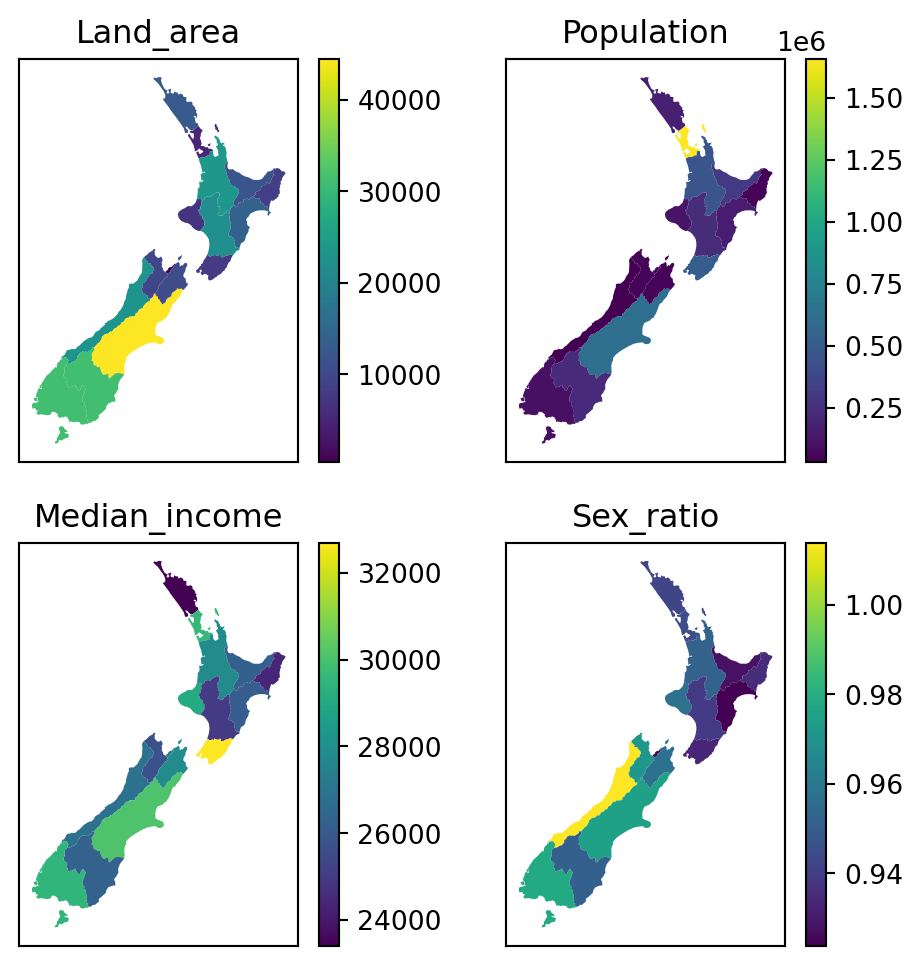Figure 8.15: 2D layout in a faceted map, using a for loop

It is also possible to “manually” specify the properties of each panel, and which row/column it goes to (e.g., Figure 2.2). This may be more useful when the various panels have different components, or even completely different types of plots (e.g., Figure 5.5), making it less practical to automate in a for loop. For example, here is the same plot as Figure 8.15, specified without using a for loop (Figure 8.16):

fig, ax = plt.subplots(ncols=2, nrows=int(len(vars)/2), figsize=(6, 6))
nz.plot(ax=ax, column=vars, legend=True)
ax.set_title(vars)
nz.plot(ax=ax, column=vars, legend=True)
ax.set_title(vars)
nz.plot(ax=ax, column=vars, legend=True)
ax.set_title(vars)
nz.plot(ax=ax, column=vars, legend=True)
ax.set_title(vars);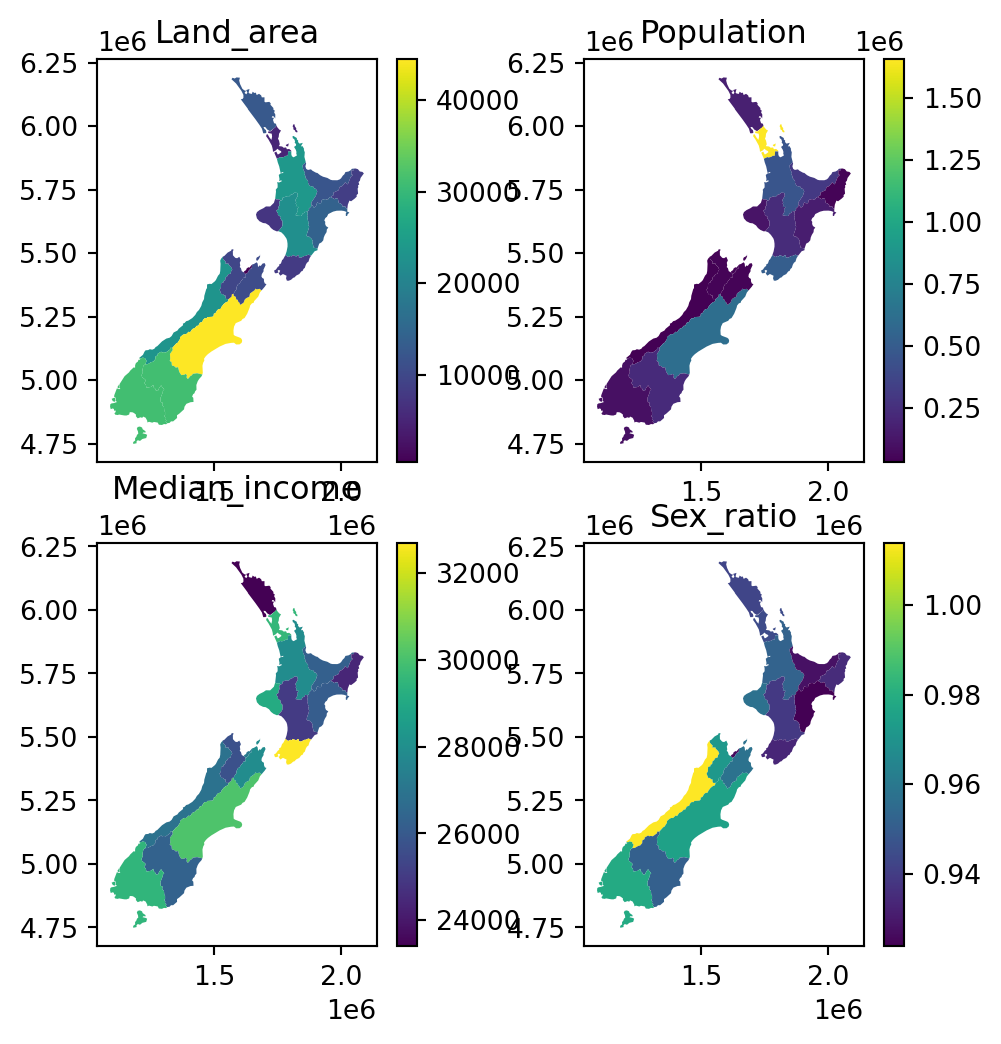Figure 8.16: 2D layout in a faceted map, using “manual” specification of the panels

See the first code section in Section 8.3.7 for another example of manual panel contents specification.

### 8.3.7 Exporting static maps

Static maps can be exported to a file using the matplotlib.pyplot.savefig function. For example, the following code section recreates Figure 7.5 (see previous Chapter), but this time the last expression saves the image to a JPG image named plot_geopandas.jpg:

fig, axes = plt.subplots(ncols=2, figsize=(9,5))
tanzania.plot(ax=axes, color='lightgrey', edgecolor='grey')
tanzania_neigh.plot(ax=axes, color='lightgrey', edgecolor='grey')
tanzania_buf.plot(ax=axes, color='none', edgecolor='red')
axes.set_title('where')
tanzania.apply(lambda x: axes.annotate(text=x['name_long'], xy=x.geometry.centroid.coords, ha='center'), axis=1)
tanzania_neigh.apply(lambda x: axes.annotate(text=x['name_long'], xy=x.geometry.centroid.coords, ha='center'), axis=1);
plt.savefig('output/plot_geopandas.jpg')

Figures with rasters can be exported exactly the same way. For example, the following code section (Section 8.3.4) creates an image of a raster and a vector layer, which is then exported to a file named plot_rasterio.jpg:

fig, ax = plt.subplots(figsize=(5, 5))
rasterio.plot.show(nz_elev, ax=ax)
nz.to_crs(nz_elev.crs).plot(ax=ax, facecolor='none', edgecolor='r');
plt.savefig('output/plot_rasterio.jpg')

Image file properties can be controlled through the plt.subplots and plt.savefig parameters. For example, the following code section exports the same raster plot to a file named plot_rasterio2.svg, which has different dimensions (width = 5 $$in$$, height = 7 $$in$$), a different format (SVG), and different resolution (300 $$DPI$$:)

fig, ax = plt.subplots(figsize=(5, 7))
rasterio.plot.show(nz_elev, ax=ax)
nz.to_crs(nz_elev.crs).plot(ax=ax, facecolor='none', edgecolor='r');
plt.savefig('output/plot_rasterio2.svg', dpi=300)

## 8.4 Interactive maps

### 8.4.1 Minimal example

An interactive map of a GeoSeries or GeoDataFrame can be created with .explore (Section 1.2.2). Here is a minimal example:

nz.explore()

### 8.4.2 Styling

The .explore method also has a color parameter, which affects both the fill and outline color. Other styling properties are specified using a dict through style_kwds (for general properties) and the marker_kwds (point-layer specific properties), as follows.

The style_kwds keys are mostly used to control the color and opacity of the outline and the fill:

• stroke—Whether to draw the outline
• color—Outline color
• weight—Outline width (in pixels)
• opacity—Outline opacity (from 0 to 1)
• fill—Whether to draw fill
• fillColor—Fill color
• fillOpacity—Fill opacity (from 0 to 1)

For example, here is how we can set green fill color and 30% opaque black outline of polygons in .explore (Figure 8.18):

nz.explore(color='green', style_kwds={'color':'black', 'opacity':0.3})

The dict passed to marker_kwds controls the way that points are displayed:

• radius—Curcle radius (in $$m$$ for circle, see below) or in pixels (for circle_marker)
• fill—Whether to draw fill (for circle or circle_marker)

Additionally, for points, we can set the marker_type, to one of:

• 'marker'—A PNG image of a marker
• 'circle'—A vector circle with radius specified in $$m$$
• 'circle_marker'—A vector circle with radius specified in pixels (the default)

For example, the following expression draws 'circe_marker’ points with 20 pixel radius, green fill, and black outline (Figure 8.19):

nz_height.explore(
color='green',
style_kwds={'color':'black', 'opacity':0.5, 'fillOpacity':0.1},
)

The following expression demonstrates the 'marker' option (Figure 8.20). Note that the above-mentioned styling properties (other then opacity) are not applicable when using marker_type='marker', because the markers are fixed PNG images:

nz_height.explore(marker_type='marker')

### 8.4.3 Layers

To display multiple layers, one on top of another, with .explore, use the m argument, which stands for the previous map (Figure 8.21):

m = nz.explore()
nz_height.explore(m=m, color='red')

One of the advantages of interactive maps is the ability to turn layers “on” and “off”. This capability is implemented in folium.LayerControl from package folium, which the geopandas .explore method is a wrapper of. For example, this is how we can add a layer control for the nz and nz_height layers (Figure 8.22). Note the name properties, used to specify layer names in the control, and the collapsed property, used to specify whether the control is fully visible at all times (False) or on mouse hover (True):

m = nz.explore(name='Polygons (adm. areas)')
nz_height.explore(m=m, color='red', name='Points (elevation)')
m

### 8.4.4 Symbology

Symbology can be specified in .explore using similar arguments as in .plot (Section 8.3.3). For example, here is an interactive version of Figure 8.6 (a).

nz.explore(column='Median_income', legend=True, cmap='Reds')

Fixed styling (Section 8.4.4) can be combined with symbology settings. For example, polygon outline colors in Figure 8.23 are styled according to 'Median_income', however, this layer has overlapping outlines and the color is arbitrarily set according to the order of features (top-most features), which may be misleading and confusing. To specify fixed outline colors (e.g., black), we can use the color and weight properties of style_kwds (Figure 8.24):

nz.explore(column='Median_income', legend=True, cmap='Reds', style_kwds={'color':'black', 'weight': 0.5})

### 8.4.5 Basemaps

The basemap in .explore can be specified using the tiles argument. Several popular built-in basemaps can be specified using a string:

• 'OpenStreetMap'
• 'Stamen Terrain'
• 'Stamen Toner'
• 'Stamen Watercolor'
• 'CartoDB positron'
• 'CartoDB dark_matter'

Other basemaps are available through the xyzservices package, which needs to be installed (see xyzservices.providers for a list), or using a custom tile server URL.

For example, the following expression displays the 'CartoDB positron' tiles in an .explore map (Figure 8.25):

nz.explore(tiles='CartoDB positron')

### 8.4.6 Exporting interactive maps

An interactive map can be exported to an HTML file using the .save method of the map object. The HTML file can then be shared with other people, or published on a server and shared through a URL. A good free option is GitHub Pages.

For example, here is how we can export the map shown in Figure 8.22, to a file named map.html:

m = nz.explore(name='Polygons (adm. areas)')
nz_height.explore(m=m, color='red', name='Points (elevation)')
m.save('output/map.html')